# 2nd PUC Basic Maths Model Question Paper 2 with Answers

Students can Download 2nd PUC Basic Maths Model Question Paper 2 with Answers, Karnataka 2nd PUC Maths Model Question Papers with Answers helps you to revise the complete Karnataka State Board Syllabus and score more marks in your examinations.

## Karnataka 2nd PUC Basic Maths Model Question Paper 2 with Answers

Time: 3.15 hours
Max. Marks: 100

Instructions

• The question paper has 5 parts A, B, C, D, and E. Answer all the parts.
• Part – A carries 10 marks, Part – B carries 20 marks, Part – C carries, Part – D carries 30 marks and Part – E carries 10 marks.
• Write the question number properly as indicated in the question paper.

Part – A

I. Answer all the questions: (10 × 1 = 10)

Question 1.
Without expanding evaluate: $$\left|\begin{array}{cc}500 & 503 \\506 & 509\end{array}\right|$$
Solution: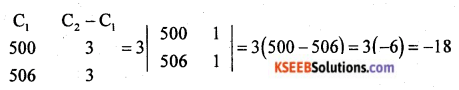Question 2.
Find r if $$^{15} \mathrm{C}_{r+3}=^{15} \mathrm{C}_{2 r-3}$$
Solution:
$$^{\mathrm{n}} \mathrm{C}_{\mathrm{r}}=^{\mathrm{n}} \mathrm{C}_{\mathrm{n}-\mathrm{r}}$$
⇒ r + (n – r) = n
⇒ (r + 3) + (2r – 3) = 15
⇒ 3r = 15
⇒ r = 5Question 3.
Negate: If Ramya study hard then she will get the rank.
Solution:
p: Ramya study hard
q: She will get the Rank
~ (P → q) = P∧~q
“Ramya study hard and she will not get the rank:”

Question 4.
If a : b = 2 : 5 and b : c = 3 : 5 find a : c.
Solution:
a : b = 2 : 5, b : c = 3 : 5
2 : 5 : 3 : 5
Multiply 1st ratio by 3 and 2nd ratio by 5
a : b & b : c
6 : 15 & 15 : 25
a : b : c = 6 : 15 : 25
a : c = 6 : 25

Question 5.
What rate of interest is realised by investing in 14.5% p.a at 81?
Solution:
For face value ₹ 100 Income = 145
₹ 81 → $$\frac{14.5 \times 81}{100}$$ = ₹ 11.75Question 6.
Find the value of sin 70° cos 20° + cos 70° sin 20°.
Solution:
Sin 70° cos 20° + cos 70° sin 20°
{∵ sin (A + B) = sin A cos B + cos A sin B [By compound angle formula]}
sin (A + B) = sin (70° + 20°) = sin 90° = 1

Question 7.
Find the equation of the point circle with centre at (4, -3).
Solution:
Point circle means radius = r = 0
Centre = (α, β) = (4, -3)
Equation of circle is (x – α)2 + (y – β)2 = r2
(x – 4)2 + (y + 3)2 = 0
Circle equation is x2 + y2 – 8x + 6y + 25 = 0

Question 8.
Evaluate $$\lim _{x \rightarrow-4} \frac{x^{4}-256}{x+4}$$
Solution: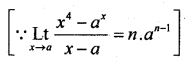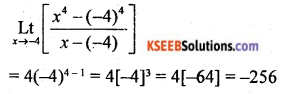Question 9.
Find $$\frac{d y}{d x}$$ if √x + √y = √a
Solut:
√x + √y = √a
Differentiate w.r.t xQuestion 10.
Evaluate $$\int\left(\frac{1+x^{2}}{x}\right) d x$$
Solution: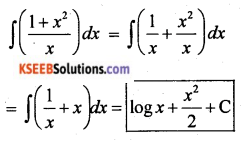Part – B

II. Answer any TEN questions: (2 × 10 = 20)

Question 11.
Prove that if any two rows (or columns) of a determinant are interchanged then the value of the determinant changes only in sign.
Solution:Question 12.
If a convex polygon has 170 diagonals, find the number of sides of the polygon.
Solution:
No. of diagonal = 170, No. of sides = n = ?
No. of diagonal = $$\frac{n(n-3)}{2}$$
170 = $$\frac{n^{2}-3 n}{2}$$
⇒ n2 – 3n = 340
⇒ n2 – 3n – 34 = 0
⇒ n2 – 20n + 17n – 340 = 0
⇒ n(n – 20) + 17(n – 20) = 0
⇒ n = 20 or n = -17
n = 20 sides [∵ n can not be -ve]

Question 13.
In a single throw of two dice, what is the probability of obtaining a total of 9?
Solution:
Total probability = $$\frac{\text { Favourable cases }}{\text { Total No. of cases }}$$ = $$\frac{4}{36}$$
P = $$\frac{1}{9}$$
sum of = [(3 + 6), (6 + 3), (4 + 5), (5 + 4)] → 4 cases
= [(3, 6) (6, 3), (4, 5), (5, 4)]Question 14.
If p, q, r are three propositions with truth values T, T, F respectively find the truth value of p → (~q ∧ r).
Solution:
p = T, q = T, r = F
p → (~q ∧ r) = T → (F ∧ F) = T → (F) = F

Question 15.
Two numbers are in the ratio 3 : 5. If 9 is subtracted from each the new numbers are in the ratio 12 : 23. Find the smaller number.
Solution:
Given ratio 3 : 5
The two are 3x and 5y
Given 9 is subtracted
$$\frac{3 x-9}{5 x-9}=\frac{12}{23}$$
⇒ 23(3x – 9) =12(5x – 2)
⇒ 69x – 207 = 60x – 108
⇒ (69x – 60x) = 207 – 108
⇒ 9x = 99
⇒ x = 11
3x = 3 × 11 = 33 → 1st no.
5x = 5 × 1 = 55 → 2nd no.

Question 16.
The Banker’s gain on a certain Bill due six months hence is ₹ 10 the rate of interest is 10% p.a. Find the face value of the Bill.
Solution:
Bankers gain = Rs. 10
Rate = 10% = 0.1, F = ?, t = $$\frac{6}{12}$$ = 0.5 yr
BG = TD (tr)
10 = T (0.5 × 0.1)
10 = TD(0.05)
TD = $$\frac{10}{0.05}$$
Again BG = BD – TD
BD = BG + TD = 10 + 200 = 210
BD = Ftr
F = BD/tr
F = $$\frac{210}{t r}=\frac{210}{0.5 \times 0.1}=\frac{210}{0.05}$$ = ₹ 4200Question 17.
If sin θ = $$\frac{3}{5}$$ and θ is acute, find the value of sin3θ.
Solution: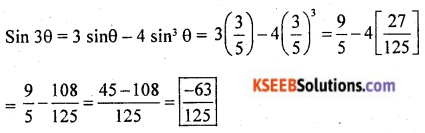Question 18.
Show that sin (A + B) sin (A – B) = sin2 A – sin2 B.
Solution:
LHS = sin (A + B) . sin (A – B)
= (sin A cos B + cos A sin B) (sin A cos B – cos A sin B)
{∵ (a + b)(a – b) = a2 – b2}
= sin2 A cos2 B – cos2 A sin2 B
= sin2 A (1 – sin2 B) – (1 – sin2 A)(sin2 B)
= sin2 A – sin2 B
= RHS

Question 19.
Find the equation of the parabola whose focus is (0, -6) and directrix y = 6.
Solution:
Focus (0, -6) = (0, a)
Directrix y = 6 ⇒ y = -a
Comapare a = -6
the required parabola equation is x2 = 4ay
x2 = 4(-6)y
x2 = -24yQuestion 20.
Find the value of k ifis continuous at x = 0.
Solution:Question 21.
If x = a sec θ, y = b tan θ find $$\frac{d y}{d x}$$
Solution: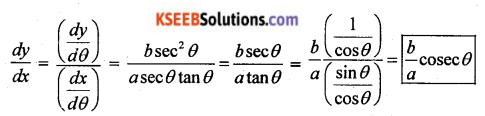Question 22.
Find a point on the parabola y2 = 18x at which ordinate increases at twice the rate of the abscissa.
Solution:
Given y2 = 18x
ordinate =y, abscissa = x,
given y = 2x
⇒ (2x)2 = 18(2x)
⇒ 4x2 = 36x
⇒ 4x(x – 9) = 0
∴ x = 0 or x = 9
∴ y = 0 or y = 18
∴ (0, 0) (9, 18) are the Two points on the parabola.

Question 23.
Find two positive numbers whose sum is 14 and the sum of the squares of the numbers is minimum.
Solution:
Let the two true nos. are x and y
Given that x + y = 14
y = 14 – x ……. (1)
Sum of the square = s = x2 + y2 = x2 + (14 – x)2
s = x2 + x2 + 196 – 28x
s = 2x2 – 28x + 196
Let f(x) = 2x2 – 28x + 196
f'(x) = $$\frac{d s}{d x}$$ = 4x – 28 = 0
⇒ 0 = 4x – 28
⇒ 4x = 28
⇒ x = 7
y = 14 – 7 = 0
Herce the Two numbers are (7, 7)Question 24.
Integrate w.r.t x $$\int\left(4 x^{2}-2 x+7\right)^{3 / 2}(4 x-1) d x$$
Solution:
put (4x2 – 2x + 7) = t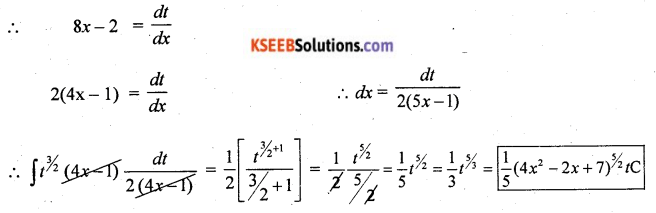Part – C

III. Answer any TEN questions (3 × 10 = 30)

Question 25.
If A = $$\left[\begin{array}{ll}2 & 3 \\4 & 5\end{array}\right]$$ show that A2 – 7A – 2I = 0. (I = Idtenity matrix of 2nd order)
Solution:
A2 – 7A – 2I = 0Question 26.
Find the adjoint of the matrix A = $$\left[\begin{array}{ccc}\cos \theta & -\sin \theta & 0 \\\sin \theta & \cos \theta & 0 \\0 & 0 & 1\end{array}\right]$$
Solution:
Cofactor of A = $$\left[\begin{array}{ccc}+(\cos \theta) & -(\sin \theta) & +(0) \\-(-\sin \theta) & +(\cos \theta) &-(0) \\+(0) & -(0) & +(1)\end{array}\right]$$
Minor of cos θ = [cos θ – 0] = cos θ
-sin θ = [sin θ – 0] = sin θ
0 = [0 – 0] = 0
sin θ = [-sin θ – 0] = -sin θ
cos θ = [cos θ – 0] = cos θ
0 = [0 – 0] = 0
0 = [0 – 0] = 0
1 = [∴ cos2 θ + sin2 θ] = 1
adjoint of A = Transpose of cofactor A = $$\left[\begin{array}{ccc}\cos \theta & \sin \theta & 0 \\-\sin \theta &\cos \theta & 0 \\0 & 0 & 1\end{array}\right]$$Question 27.
In how many ways 3 boys and 5 girls can be arranged in a row so that no two boys are together?
Solution:
No two boys together, first we have to arrange 5 girls in $$^{5} \mathrm{P}_{5}$$ = 5!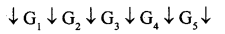There are 6 place between 5 girls where we can arrange 3 boys = $$^6 \mathbf{P}_{3}$$ ways
Total no. of arrangement = 5! × $$^{6} \mathrm{P}_{3}$$

Question 28.
If A and B are events with P(A) = $$\frac{5}{8}$$, P(B) = $$\frac{3}{8}$$ and P(A∪B) = $$\frac{3}{4}$$. find (i) P(B/A) (ii) P(A/B)
Solution: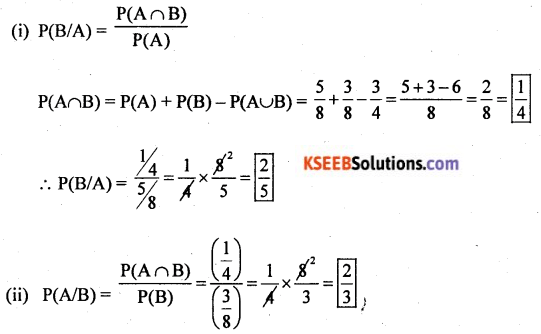Question 29.
Two trains 130m and 80m long are running in opposite directions and are at the rate of 75km/hr and 33km/hr respectively. From the moment they meet find the time of the two trains cross each other?
Solution:
If they are running in opposite directions, their relative speed will be 75 + 33 = 118 km per hour.
Time Taken = $$\frac{\text { Distance travelled }}{\text { speed }}$$ = $$\frac{210}{118}$$ = 1.78 hourQuestion 30.
A banker pays ₹ 2380 on a bill of ₹ 2500, 73 days before the legally due date. Find the rate of discount charged by the banker.
Solution:
F = ₹ 2500
Discount value of the bill = ₹ 2380BD = F – DVB = ₹ 2500 – ₹ 2380 = ₹ 120
Ftr = BD
Ftr = 120
r = $$\frac{120}{\mathrm{Ft}}$$
Rate of discount = r = $$\frac{120}{2500 \times 0.2}=\frac{12}{500}$$ = 0.24 of 24%

Question 31.
Find the interest earned on ₹ 4897.50 cash invested in 15% stock at 81.5 the brokerage given is 0.125.
Solution:
Each stock value = 81.5Question 32.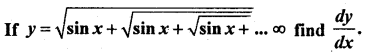Solution: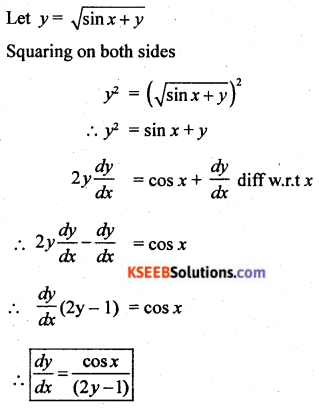Question 33.
Find the co-ordinates of the vertex, focus and equation of directrix of the parabola 5x2 + 24y = 0.
Solution:
Given parabola 5x2 + 24y = 0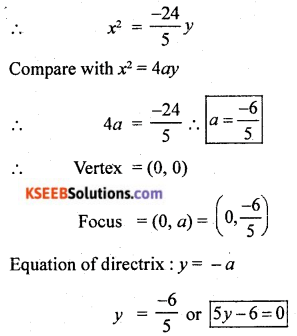Question 34.
Raju goes to purchase a motorcycle which is priced ₹ 35,640 including 10% on sales tax however the actual rate of sales tax at the time of purchase is 7%. Find the extra profit made by the shop keeper if he still charges the original listed price.
Solution:
Let the marked price = ₹ x
S.P = ₹ 35,640
S.T = 10%
Total amount to be paid = M.P + ST% of MP
35,640 = x + 10% of x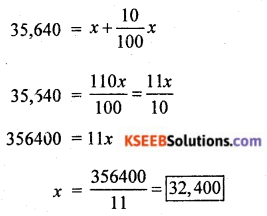At the time of purchase the S.T is 7%
Raju paid at the purchase time = $$\frac{32400 \times 7}{100}$$ = 2,268
SP = 32,400 + 2,268 = ₹ 34,557
Profit by the shop keeper = (35,640) – (34,668) = ₹ 972Question 35.
A circular plate of metal is heated so that its radius increases at the rate of 0.1 mm per minute. At what rate is the plate’s area increasing when the radius is 25 cm.
Solution: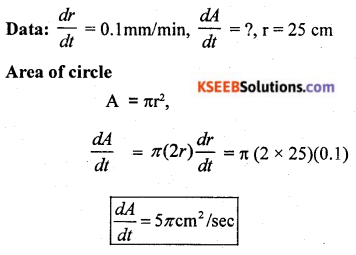Question 36.
The cost function C = 500x – 20x2 + $$\frac{x^{3}}{3}$$ where x stands for output. Calculate the output when the marginal cost is equal to average cost.
Solution:
C = 500x – 20x2 + $$\frac{x^{3}}{3}$$
Marginal cost = Average cost,
x = ?Question 37.
Evaluate $$\int \frac{1}{e^{x}+e^{-x}} d x$$
Solution: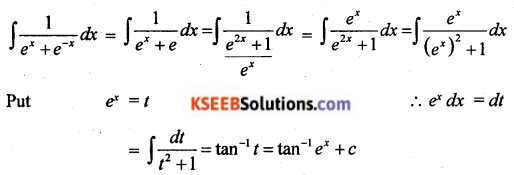Question 38.
Evaluate: $$\int_{0}^{\pi / 2} \sin 5 x \cdot \cos 3 x d x$$
Solution:
$$\int_{0}^{\pi / 2} \sin 5 x \cdot \cos 3 x d x$$
By using transformation formulae of production in to sum is
sin A cos B = $$\frac{1}{2}$$ (sin (A + B) + sin (A – B))Part – D

IV. Answer any SIX questions: (5 × 6 = 30)

Question 39.
Find the coefficient of x-11 in the expansion of $$\left(\sqrt{x}-\frac{2}{x}\right)^{17}$$
Solution: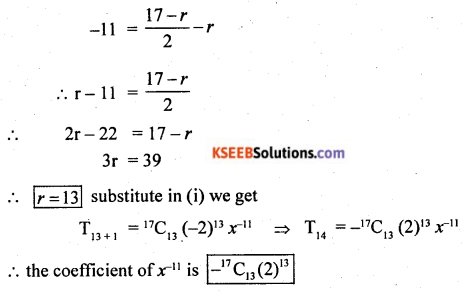Question 40.
Resolve $$\frac{2 x^{2}+16 x+29}{(x+3)^{2}(x+4)}$$ into partial fractions.
Solution:
$$\frac{2 x^{2}+16 x+29}{(x+3)^{2}(x+4)}=\frac{A}{(x+4)}+\frac{B}{(x+3)}+\frac{C}{(x+2)^{2}(x+3)^{2}}$$
2x2 + 16x + 29 = A(x + 3)2 + B(x + 4)(x + 3) + C(x + 4)
put x = -4
2(-4)2 + 16(-4) + 29 = A(-4 + 3)2 + 0 + 0
⇒ 32 – 64 + 29 = A(1) + 0 + 0
⇒ -2 = A
⇒ A = -2
Put x = -3
2(-3)2 + 16(-3) + 29 = 0 + 0 + C(-3 + 4)
⇒ 18 – 48 + 29 = C(1)
⇒ -1 = C
⇒ C = -1
Equate the coefficient of x2 on both side
2 = A + B
⇒ 2 = -2 + B
⇒ B = 4
$$\frac{2 x^{2}+16 x+29}{(x+4)(x+3)^{2}}=\frac{-2}{(x+4)}+\frac{4}{(x+3)}-\frac{1}{(x+3)^{2}}$$ are partial fractionsQuestion 41.
Prove that, ~(p ↔ q) = (p ∧ ~q) ∨ (q ∧ ~p).
Solution: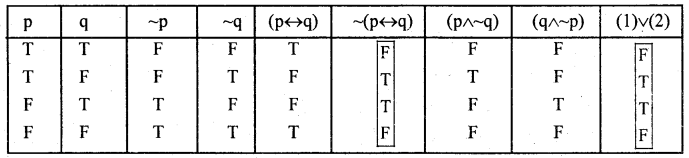LHS = RMS from 6th column and 9th column

Question 42.
If 15 men working 12 hours per day perform the job in 16 days how long will it take for 21 men working 10 hours daily to do the same job?
Solution: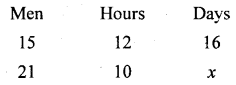More men → less days (Inverse propertion)
15 : 21 = x : 16 ….(1)
More hours → Less days (Inverse proportion)
12 : 10 = x : 16 ……(2)
From (1) and (2)
(15 × 12) : (21 × 10) = x : 16
⇒ $$\frac{180}{210}$$ = $$\frac{x}{16}$$
⇒ 7x = 96
⇒ x = $$\frac{96}{7}$$ = 13.7 days

Question 43.
The time required to produce the first unit of a product is 1000 hours. If the manufactures experience 80% learning effect. Calculate the cumulative average time per unit and the total time taken to produce altogether 8 units. Also, find the labour charges for the production of 8 units at the rate of ₹ 10 per hour.
Solution:Total labour hours to produce 8 unit = 4096 hr
Total labour cost at ₹ 10/hr = 4096 × 10 = ₹ 40,960Question 44.
Solve L.P.P graphically, maximize z = 4x + 3y subject to the constraints x + 2y ≤ 5, x + y ≤ 3, 3x + y ≤ 7, x, y ≥ 0.
Solution: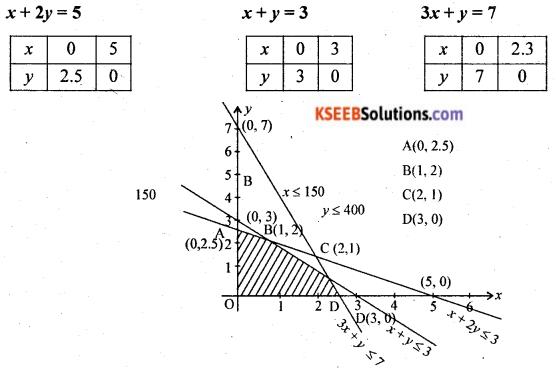at A(0, 2.5), z = 4(0) + 3(2.5) = 7.5
at B (1, 2), z = 4(1) + 3(2) = 10
at C (2, 1), z = 4(2) + 3(1) = 11
at D (3, 0), z = 4(3)+ 3(0) = 12
z is maximise at D (3, 0)
∴ x = 3, y = 0

Question 45.
The angle of elevation of an object from a point 100 m above a lake is 30° and angle of depression of its image in the lake is 45°. Find the height of the object above the lake.
Solution: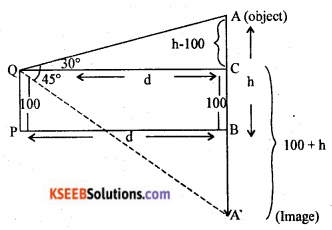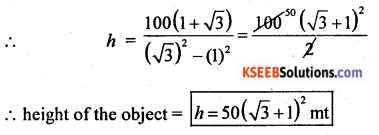Question 46.
Find the equation of the circle possing through the points (1, 1), (2, -1) and (3, 2).
Solution:
Let the equation of circle be x2 + y2 + 2gx + 2fy + C = 0 ……. (1)
This equation passes through (1, 1) (2, -1) and (3, 2)
at (1, 1), 1 + 1 + 2g + 2f + C = 0
2g + 2f + C + 2 = 0 …… (2)
at (2, -1), 4 + 1 + 4g – 2f + C = 0
4g – 2f + C + 5 = 0 …… (3)
at (3, 2), 9 + 4 + 6g + 4f + C = 0
6g + 4f + C + 13 = 0 …… (4)(1) becomes x2 + y2 – 5x – y + 4 = 0 is the circle equationQuestion 47.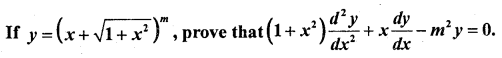Solution:
Given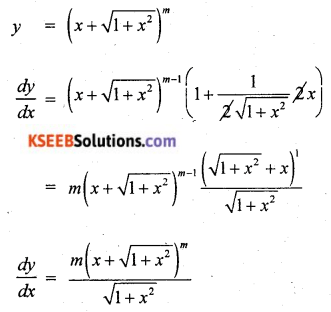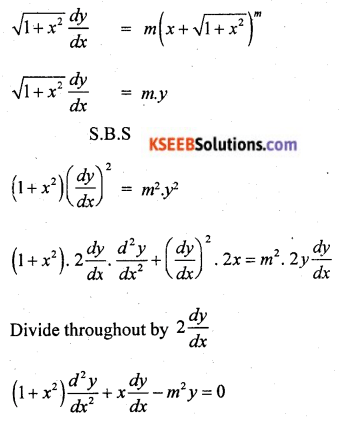Question 48.
Find the area enclosed between the curves y2 = 4x and x2 = 4
Solution:
x2 = 4y ⇒ y = $$\frac{x^{2}}{4}$$
y2 = 4x
⇒ $$\left(\frac{x^{2}}{4}\right)^{2}$$ = 4x
⇒ x4 = 64
⇒ x(x3 – 43) = 0
⇒ x = 0 or x = 4 are limits of integration
Required Area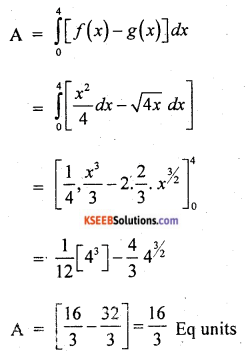Part – E

V. Answer any one question: (1 × 10 = 10)

Question 49.
(a) Salesman Venki has the following record of sales during 3 months of July, August and September for three products. A, B, C which have different rates of commission.Using matrix method, find out the rates of commission on items A, B and C received by Venki. (6)
Solution:
Consider, 100x + 100y + 100z = 700
200x + 300y + 200z = 1700
400x + 900y + 100z = 3700
÷ by 100
x + y + z = 7
2x + 3y + 2z = 17
4x + 9y + z = 37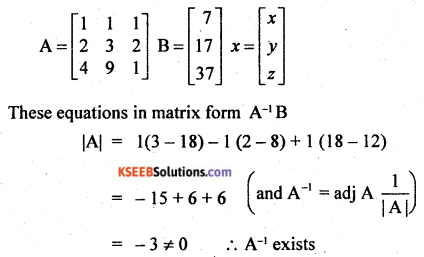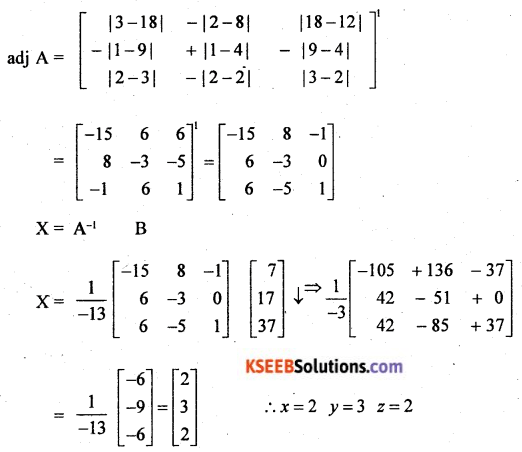(b) Find the value of (0.98)3 using binomial theorem up to 5 places of decimals. (4)
Solution:
(0.98)3 = (1 – 0.02)3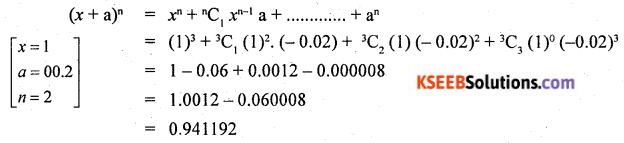Question 50.
(a) Prove that $$\lim _{\theta \rightarrow 0} \frac{\sin \theta}{\theta}=1$$ and hence deduce that $$\lim _{\theta \rightarrow 0} \frac{\tan \theta}{\theta}=1$$. (θ in radian) (6)
Solution:
Let ‘O’ the centre of unit circle, assume that θ is measured in positive radians
Let ∠AOB = θc, from A, draw AD is ⊥ to OA, meeting OB produced to D, therefore OA = OB = radians
From the diagram, we have an area of ∆AOB = area of sector AOB = area of ∆AOD
area of ∆AOB ≤ area of sector ∆AOB ≤ area of ∆AOD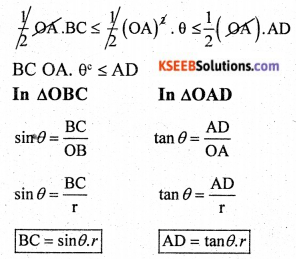(b) A company produces two types of leather belts A and B. A is of superior quality and B is of inferior quality. The respective profits are ₹ 10 and ₹ 5 per belt. The supply of raw material is sufficient for making 850 belts per day. For belt A, a special type of buckle is required and 500 are available per day. There are 700 buckles available for belt B per day. Belt A needs twice as much time as that required for belt B and the company can produce 500 belts if all of them were of the type A. Formulate an L.P.P model for the above problem. (4)
Solution:
Objective function max z = 10x + 5y
Subject to constrains
2x + y = 500
x ≥ 0, y ≥ 0

error: Content is protected !!